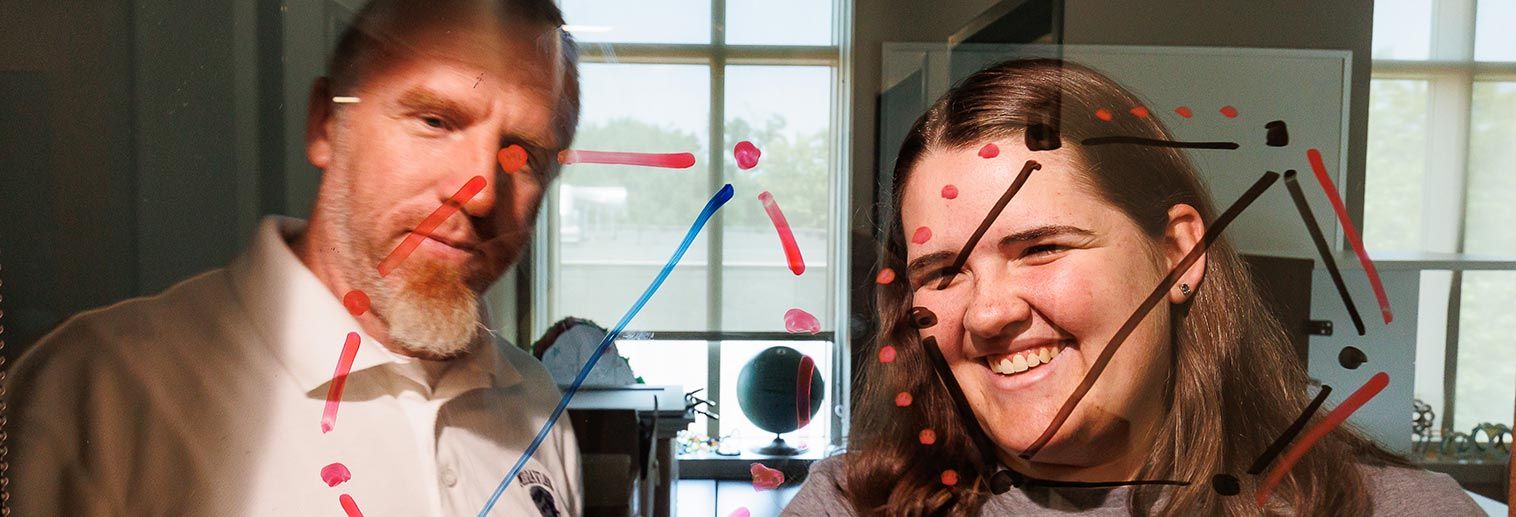# Applied Mathematics Track

Students majoring in Applied Mathematics will develop the strong math foundation required to solve real-world problems. This is ideal for students interested in business, industry, or graduate school in applied mathematics.

## Curriculum Requirements

The following courses are required for all tracks:

• Calculus I (MATH 170) or equivalent*
• Calculus II (MATH 171)
• Calculus III (MATH 211)
• Discrete Math/Proofs (MATH 212)
• Mathematics Seminar (MATH 370)

*Or equivalent MATH 106, 166 sequences

The Applied Mathematics track also requires the following:

• Linear Algebra (MATH 220)
• Differential Equations (MATH 254)
• One Pure Math course
• Three Applied Math courses
• Choose two Corequisite Courses:
• Biostatistics (BIOL 220)
• Computer Science I (CSCI 120)
• Computer Science II (CSCI 121)
• Inter Microeconomics (ECON 225)
• Applied Econometrics (ECON 256)
• Physics I (PHYS 111)
• Physics II (PHYS 112)

### Course Options

For the purposes of flexibility and degree customization, the Applied Mathematics track allows students to round out their schedule with the choice of one Pure Math course and three Applied Math courses from the lists below. At least three (3) courses must be 310-380, 390-399, or 400-401.

Pure Math Courses:

• Modern Algebra (MATH 347)
• Higher Geometry (MATH 348)
• Complex Analysis (MATH 365)
• Introduction to Analysis (MATH 366)

Applied Math Courses:

• Operations Research (MATH 230)
• Mathematical Statistics I (MATH 231)
• Applied Statistics (MATH 232)
• Actuarial Mathematics (MATH 251)
• Numerical Analysis (MATH 258)
• Mathematical Statistics II (MATH 337)
• Applied Statistics Modeling (MATH 338)
• Mathematical Physics (PHYS 343)

“Differential Equations [was my favorite class]. I loved to be able to apply it. I liked that when I would see things in other classes that were related to differential equations. This happened a lot. This really opened my mind to the fact that mathematics has a lot of overlap between classes. Being able to always learn something in other classes is mind-blowing.”

—Alvaro Belmonte ’20

## Interested in Teaching?

### Secondary Education Certification

Pure Mathematics track students who are planning to teach mathematics in secondary schools must complete the following courses: MATH 170 (or its equivalent sequence MATH 106 and  MATH 166), 171, 211, 212, 220, 232, 347, 348, 370, and one additional pure mathematics course numbered 210 or above.

### Middle-Level Education Certification

Students who are seeking certification in middle-level education with a major in mathematics can complete either the Pure or Applied Mathematics Track.

### Early Childhood Education Certification

Students who are seeking certification in early childhood education with a major in mathematics should take the Pure Mathematics Track and are required to complete PHYS 111. The second co-requisite course is waived for these students. Students who are pursuing early childhood teacher certification with a major in mathematics do not need to complete MATH 120.